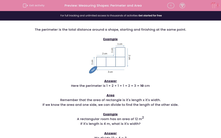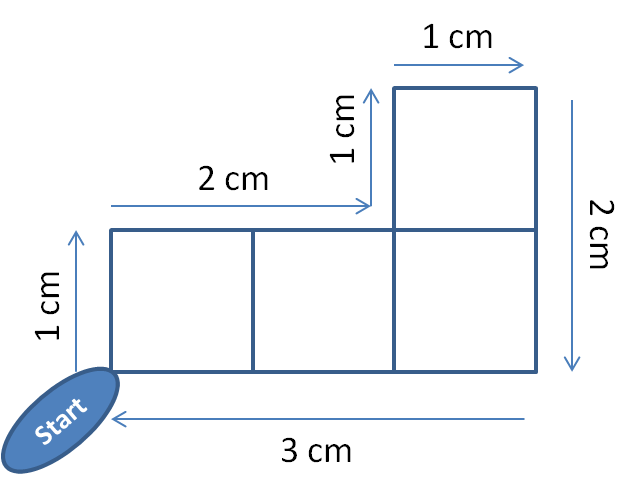# Measuring Shapes: Perimeter and Area

In this worksheet, students answer questions on area and perimeter.This content is premium and exclusive to EdPlace subscribers.Key stage:  KS 2

Curriculum topic:   Maths and Numerical Reasoning

Curriculum subtopic:   Perimeter and Area

Difficulty level:#### Worksheet Overview

The perimeter is the total distance around a shape, starting and finishing at the same point.

ExampleHere the perimeter is 1 + 2 + 1 + 1 + 2 + 3 = 10 cm

Area

Remember that the area of rectangle is it's length x it's width.

If we know the area and one side, we can divide to find the length of the other side.

Example

A rectangular room has an area of 12 m2

If it's length is 4 m, what is it's width?